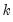Command Reference : Command Reference

 liml
Limited Information Maximum Likelihood and K-class Estimation.
Syntax
liml(options) y c x1 [x2 x3 ...] @ z1 [z2 z3 ...]
liml(options) specification @ z1 [z2 z3 ...]
To use the liml command, list the dependent variable first, followed by the regressors, then any AR or MA error specifications, then an “@”-sign, and finally, a list of exogenous instruments.
You may estimate nonlinear equations or equations specified with formulas by first providing a specification, then listing the instrumental variables after an “@”-sign. There must be at least as many instrumental variables as there are independent variables. All exogenous variables included in the regressor list should also be included in the instrument list. A constant is included in the list of instrumental variables, unless the noconst option is specified.
Options

 noconst Do not include a constant in the instrumental list. Without this option, a constant will always be included as an instrument, even if not specified explicitly. w=arg Weight series or expression. wtype=arg (default=“istdev”) Weight specification type: inverse standard deviation (“istdev”), inverse variance (“ivar”), standard deviation (“stdev”), variance (“var”). wscale=arg Weight scaling: EViews default (“eviews”), average (“avg”), none (“none”).The default setting depends upon the weight type: “eviews” if “wtype=istdev”, “avg” for all others. kclass=number Set the value ofin the K‑class estimator. If omitted, LIML is performed, andis calculated as part of the estimation procedure. se = arg (default=“iv”) Set the standard-error calculation type: IV based (“se=iv”), K-Class based (“se=kclass”), Bekker (“se=bekk”), or Hansen, Hausman, and Newey (“se=hhn”). m=integer Set maximum number of iterations. c=number Set convergence criterion. The criterion is based upon the maximum of the percentage changes in the scaled coefficients. The criterion will be set to the nearest value between 1e-24 and 0.2. numericderiv / ‑numericderiv [Do / do not] use numeric derivatives only. If omitted, EViews will follow the global default. fastderiv / ‑fastderiv [Do / do not] use fast derivative computation. If omitted, EViews will follow the global default.Available only for legacy estimation (“optmeth=legacy”). showopts / ‑showopts [Do / do not] display the starting coefficient values and estimation options in the estimation output. coef=arg Specify the name of the coefficient vector (if specified by list); the default behavior is to use the “C” coefficient vector. prompt Force the dialog to appear from within a program. p Print estimation results.
Examples
liml gdp c cpi inc @ lw lw(-1)
calculates a LIML estimation of GDP on a constant, CPI, and INC, using a constant, LW, and LW(-1) as instruments.
Cross-references
See “Limited Information Maximum Likelihood and K-Class Estimation” for discussion.
See Equation::liml for the equivalent equation object method.